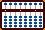###Convert Hex Numbers to Binary and Add

Here's an easy and effective method for adding hexadecimal (base 16) numbers using the binary (base 2) number system. The method involves converting the hexadecimal numbers to binary numbers which are then added together. When the sums are completed the binary answer is converted back to hexadecimal.

What is binary? How does it work?

The binary number system is a base 2 number system which has only two values; 0 and 1. It's the number system used internally by almost all modern computers. Binary numbers can easily be placed onto the soroban by either leaving a rod empty or by adding one lower (earth) bead; 101101.

When converting a binary number like 101101 into a hexadecimal number it's a good idea to separate the binary numbers into groups of four rods each. It helps to make the hexadecimal numbers more recognizable. Start from the right and work left counting groups of four. Whenever the binary numbers can't group into four 10,1101 add zeros to make up the difference 0010,1101. Consult the Hexadecimal and Binary Equivalents table below and convert. This hexadecimal group 0010,1101 reads 2D

Two things we need to know before we begin to add in binary.

1) Binary equivalents

We need to know the binary number equivalents of hex 1 through F. Use your abacus and count to see the pattern. If you can count binary 1, 2 and 3 on your abacus you're on your way because the pattern repeats over and over. It's that simple.

 1 = 0001 2 = 0010 3 = 0011 4 = 0100 5 = 0101 6 = 0110 7 = 0111 8 = 1000 9 = 1001 A = 1010 B = 1011 C = 1100 D = 1101 E = 1110 F = 1111

2) Normalize a Binary Number

We need to know how to normalize a binary number. Because binary numbers only have two values (0 and 1) we need to normalize a number when it's value is greater than 1. Normalizing is done by carrying 1 to the left for every 2 we have on any given rod.

For Example: After adding binary numbers on our abacus we could end up with the following. Because they aren't in binary form we have to normalize them to make them binary numbers.

0102 would normalize to binary 0110
0003 would normalize to binary 0011
0004 would normalize to 0012, then 0020 and finally to binary 0100
0202 would normalize to 1002. then finally to binary 1010

Example 1: Add binary 0001 + 0011 = 0100 (in base 10 and base 16 this is 1+3 = 4)

abcd
0001 : set 0001
= 0012 : Here we have to normalize because we can't have a value of more than 1 on any rod.

0020 : normalize the 2 on rod d
0100 : normalize the 2 on rod c and the answer is 0100, which is 4 in hexadecimal.

In order to add hexadecimal numbers consult the 'Hex Binary Equivalents table' and convert the hex numbers to binary. Then simply add the binary numbers together and convert back to hexadecimal.

Example 2: Add hex A+A = 14. Use the table and convert hex A to binary. In binary the problem is 1010 + 1010 = 10100

abcdefgh
00001010
+ 00001010
= 00002020 : not normalized

00010020 : normalize the 2 on e
00010100 : normalize the 2 on g and we have the binary answer 10100. Now convert this to hex;

The answer: Starting from the right and working left, separate the binary number 10100 into groups of four 0001,0100 which is 14 hex.

Example 3: Add hex 9 + 3 = C. In binary the problem is 1001 + 0011 = 1100

abcdefgh
00001001
+ 00000011
= 00001012 : not normalized

00001020 : normalize the 2 on h
00001100 : normalize the 2 on g and we have the binary answer 1100.

Example 4: Add hex A+B+C= 21. In binary the problem is 1010 + 1011 + 1100 = 100001

abcdefgh
00001010
00001011
+ 00001100
= 00003121 : not normalized

00011121 : normalize 3 on e
00011201 : normalize 2 on g
00012001 : normalize 2 on f
00020001 : normalize 2 on e
00100001 : and finally normalize 2 on d and we have the binary answer 100001. Now convert this to hex;

The answer: Working right to left, separate the binary number 100001 into groups of four digits 0010,0001 which is 21 hex.

Example 5: Add hex 21 + 3B = 5C. In binary the problem is 100001 + 111011 = 1011100

abcdefgh
00100001
+ 00111011
= 00211012 : not normalized

01011020 : normalize 2 on c
01011100 : normalize 2 on g and we have the binary answer 1011100. Now convert this to hex;

The answer: Working right to left, separate the binary number 1011100 into groups of four digits 0101,1100 which is 5C.

This is such an easy way to add hexadecimal numbers in part because all we're doing is adding the binary numbers 1 and 0. Normalizing a number is a little tricky at first but in the end even that is simple enough to learn. The really great thing is that this technique can be done on any kind of abacus, be it a Japanese 1:4 bead soroban or Chinese 2:5 bead suan pan.